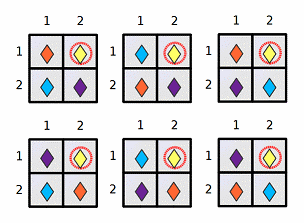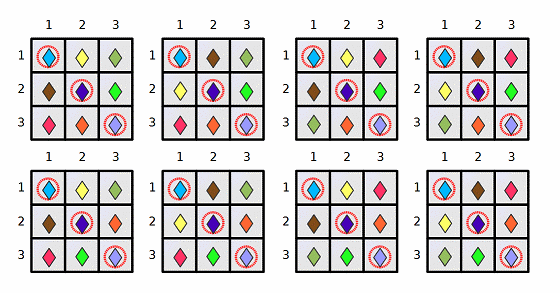### Problem Statement

Fox Ciel has a rectangular board separated into 1x1 cells. The board is N cells wide and M cells high. Columns are numbered 1 to N from left to right, and rows are numbered 1 to M from top to bottom. A cell in column x, row y is said to have coordinates (x, y). Each cell contains a stone, and all stones are different. Also, some cells are marked. These marked cells are given in the int[]s sx and sy, where (sx[i], sy[i]) are the coordinates of the i-th marked cell.

Ciel is interested to know how many layouts of the same stones on this board are similar to the current layout. Two layouts are considered to be similar if, for each possible pairing of a stone and a marked cell, the distance between the stone and the marked cell is the same in both layouts. The distance between cells with coordinates (xA, yA) and (xB, yB) is defined as max{|xA-xB|, |yA-yB|}, where |z| is the absolute value of z.

Return the number of layouts that are similar to the current layout, modulo 1,000,000,009. Note that according to the definition above, the current layout is similar to itself, so it should also be counted.

### Definition

 Class: FoxStones Method: getCount Parameters: int, int, int[], int[] Returns: int Method signature: int getCount(int N, int M, int[] sx, int[] sy) (be sure your method is public)

### Constraints

-N and M will each be between 1 and 200, inclusive.
-sx and sy will each contain between 1 and 50 elements, inclusive.
-sx and sy will contain the same number of elements.
-Each element of sx will be between 1 and N, inclusive.
-Each element of sy will be between 1 and M, inclusive.
-No two cells represented by sx and sy will have the same coordinates.

### Examples

0)

 `6` `1` `{3}` `{1}`
`Returns: 4`
 There are 4 similar layouts:1)

 `2` `2` `{2}` `{1}`
`Returns: 6`2)

 `3` `3` `{1,2,3}` `{1,2,3}`
`Returns: 8`3)

 `12` `34` `{5,6,7,8,9,10}` `{11,12,13,14,15,16}`
`Returns: 410850247`

#### Problem url:

http://www.topcoder.com/stat?c=problem_statement&pm=11316

#### Problem stats url:

http://www.topcoder.com/tc?module=ProblemDetail&rd=14427&pm=11316

ir5

#### Testers:

PabloGilberto , ivan_metelsky , pieguy

Math